# Java Program to Calculate Average Using Arrays

In this tutorial you will learn about the Java Program to Calculate Average Using Arrays and its application with practical example.

In this tutorial, we will learn to create a Java program that will Calculate Average Using Arrays   using Java programming.

## Prerequisites

Before starting with this tutorial we assume that you are best aware of the following Java programming topics:

• Java Operators.
• Basic Input and Output
• Class and Object.
• Basic Java programming.
• Array.

## What is array?

An array is  a derived Data type that holds a fixed number of values of a single Type. An Array is a collection variable elements  that can store multiple values in a single variable of same type.In Computer Science an array is a data structure, or simply an array, is a data structure consisting of a collection of elements.

## Java Program to Calculate Average Using Arrays

In this program we will find average of an array elements. We would first declared and initialized the required variables. Next, we would prompt user to input elements of array .Later we add all the elements and find Average of Array elements.

## Output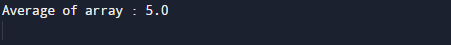In the above program, we have first declared and initialized a set variables required in the program.

• a[] = it will hold entered elements.
• sum =hold sum of values.
• i = for iteration.
• avg= it will hold average of numbers.

First of all we initialize value in array.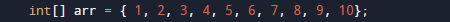After that we will find the length of an array. and value of sum is initialize to zero.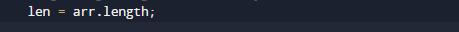then using for loop we will add all the values of an array in variable sum .To calculate the Average we need to first calculate the sum of all elements in the array.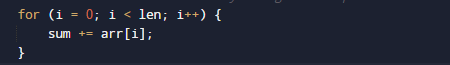After that we will add elements of an array.Finally, we calculate the average.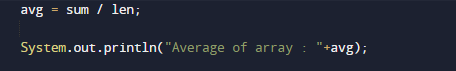In this tutorial we have learn about the Java Program to Calculate Average Using Arrays and its application with practical example. I hope you will like this tutorial.